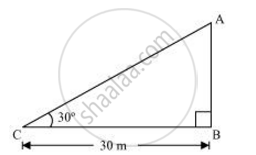Share

# The angle of elevation of the top of a tower from a point on the ground, which is 30 m away from the foot of the tower, is 30°. Find the height of the tower - Mathematics

Course

#### Question

The angle of elevation of the top of a tower from a point on the ground, which is 30 m away from the foot of the tower, is 30°. Find the height of the tower

#### SolutionLet AB be the tower and the angle of elevation from point C (on ground) is

30°.

In ΔABC,

(AB)/(BC) = tan 30^@

(AB)/30 = 1/sqrt3

AB = 30/sqrt3 = 10sqrt3 m

Therefore, the height of the tower is 10sqrt3 m

Is there an error in this question or solution?